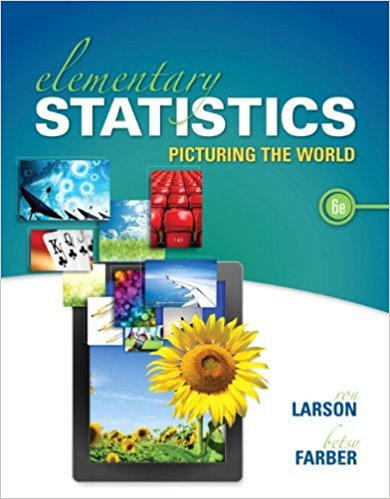×
Get Full Access to Elementary Statistics: Picturing The World - 6 Edition - Chapter 4.2 - Problem 13e
Get Full Access to Elementary Statistics: Picturing The World - 6 Edition - Chapter 4.2 - Problem 13e

×

# Answer: Identifying and Understanding Binomial ExperimentsISBN: 9780321911216 66

## Solution for problem 13E Chapter 4.2

Elementary Statistics: Picturing the World | 6th Edition

• Textbook Solutions
• 2901 Step-by-step solutions solved by professors and subject experts
• Get 24/7 help from StudySoup virtual teaching assistantsElementary Statistics: Picturing the World | 6th Edition

4 5 1 296 Reviews
25
4
Problem 13E

Identifying and Understanding Binomial Experiments In Exercise, determine whether the experiment is a binomial experiment. If it is, identify a success, specify the values of n, p, and q, and list the possible values of the random variable x. If it is not a binomial experiment, explain why.Lottery A state lottery randomly chooses 6 balls numbered from 1 through 40 without replacement. You choose six numbers and purchase a lottery ticket. The random variable represents the number of matches on your ticket to the numbers drawn in the lottery.

Step-by-Step Solution:

Solution:

Step 1 of 2:

We have to identify whether the given experiment is binomial or not.

Six balls are randomly selected from a group of balls numbered from 1 through 40 without replacement.

The random variable x represents the number of matches on your ticket to the numbers drawn in the lottery.

We also want to find the values of n, p, and q, and the possible values of the random variable, if it is binomial experiment.

Step 2 of 2

##### ISBN: 9780321911216

Elementary Statistics: Picturing the World was written by and is associated to the ISBN: 9780321911216. This full solution covers the following key subjects: lottery, binomial, experiment, ticket, values. This expansive textbook survival guide covers 66 chapters, and 3049 solutions. The answer to “Identifying and Understanding Binomial Experiments In Exercise, determine whether the experiment is a binomial experiment. If it is, identify a success, specify the values of n, p, and q, and list the possible values of the random variable x. If it is not a binomial experiment, explain why.Lottery A state lottery randomly chooses 6 balls numbered from 1 through 40 without replacement. You choose six numbers and purchase a lottery ticket. The random variable represents the number of matches on your ticket to the numbers drawn in the lottery.” is broken down into a number of easy to follow steps, and 89 words. This textbook survival guide was created for the textbook: Elementary Statistics: Picturing the World , edition: 6. The full step-by-step solution to problem: 13E from chapter: 4.2 was answered by , our top Statistics solution expert on 08/25/17, 09:43AM. Since the solution to 13E from 4.2 chapter was answered, more than 567 students have viewed the full step-by-step answer.

Unlock Textbook Solution